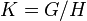# Commuting fraction in quotient group is at least as much as in whole group

## Statement

Suppose$G$ is a finite group and$H$ is a normal subgroup. Let$K = G/H$ be the quotient group. Then, the following are true:

1. The Commuting fraction (?) (i.e., the fraction of pairs of elements that commute) in$K$ is at least as much as in$G$.
2. The Number of conjugacy classes (?) in$K$ is bounded from below by the quotient of the number of conjugacy classes in$G$ by the size of$H$.